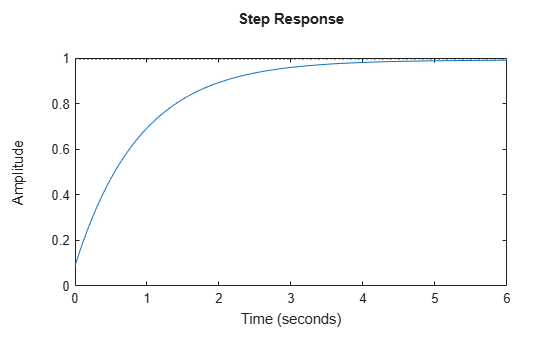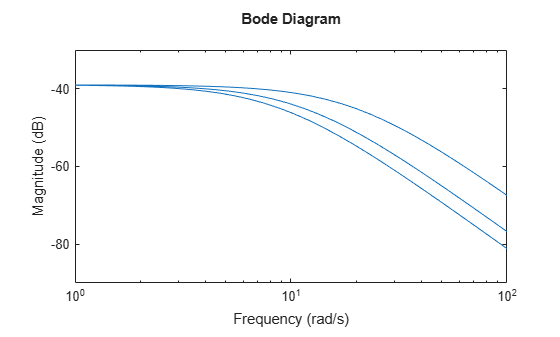# sample

Sample linear parameter-varying or time-varying dynamics

Since R2023a

## Syntax

``ssArray = sample(vSys,T)``
``ssArray = sample(vSys,T,P)``
``ssArray = sample(vSys,T,P1,...,Pn)``
``ssArray = sample(vSys,S)``
``ssArray = sample(vSys)``
``[ssArray,offsets] = sample(___)``

## Description

example

````ssArray = sample(vSys,T)` samples the dynamics of the linear time-varying (LTV) model `vSys` at the times specified in the time vector `T` and returns an array of time-invariant state-space (`ss`) models. ```

example

````ssArray = sample(vSys,T,P)` samples the dynamics of the linear-parameter varying (LPV) model `vSys` at the single point (`T`,`P`) and returns an array of time-invariant state-space (`ss`) models. Set `T` to `[]` if the dynamics of the LPV model only depend on `P`.```
````ssArray = sample(vSys,T,P1,...,Pn)` samples the dynamics over a grid of (`T`,`P`) values. `P1,...,Pn` are arrays specifying the values of each parameter of the LPV model.```

example

````ssArray = sample(vSys,S)` specifies the sample values as a structure `S`.```

example

````ssArray = sample(vSys)` samples a gridded LTV or LPV model at values obtained from `vSys.Grid`. You can use this syntax as a quick way to access the state-space data and offsets used to construct the gridded LTV or LPV model.```

example

````[ssArray,offsets] = sample(___)` also returns a structure array `offsets` containing the derivative, state, input, and output offset values at the specified times. You can use this syntax with any of the input-argument combinations in previous syntaxes.```

## Examples

collapse all

You can sample the dynamics of an LTV model over a point or a vector of $\mathit{t}$ values to obtain affine dynamics for a given time.

Consider a model defined by the data function `ltvssDataFcn.m`.

Create an LTV model.

`ltvSys = ltvss(@ltvssDataFcn)`
```Continuous-time state-space LTV model with 1 outputs, 1 inputs, and 1 states. ```

Define a set of times values to sample this model over.

`t = 5:0.5:10;`

Use the `sample` command to obtain an array of `ss` models.

```[ssArray,offsets] = sample(ltvSys,t); size(ssArray)```
```1x11 array of state-space models. Each model has 1 outputs, 1 inputs, and 1 states. ```

Plot the step response of the fourth model in the array.

```x0 = offsets(4).x; respOpt = RespConfig(Amplitude=1,InitialState=x0); step(ssArray(:,:,:,4),respOpt)```View the data function.

`type ltvssDataFcn.m`
```function [A,B,C,D,E,dx0,x0,u0,y0,Delays] = ltvssDataFcn(t) % SISO, first order A = -(1+0.5*sin(t)); B = 1; C = 1; D = 0; E = []; dx0 = []; x0 = []; u0 = []; y0 = 0.1*sin(5*t); Delays = []; ```

You can sample the dynamics of an LPV model over a point or a grid of ($\mathit{t}$,$\mathit{p}$) values to obtain affine dynamics for a given time or parameter value.

For this example, `dataFcnMaglev.m` defines the matrices and offsets of a magnetic levitation system. The magnetic levitation controls the height of a levitating ball using a coil current that creates a magnetic force on the ball.

Create an LPV model.

`lpvSys = lpvss('h',@dataFcnMaglev)`
```Continuous-time state-space LPV model with 1 outputs, 1 inputs, 2 states, and 1 parameters. ```

Sample the LPV dynamics at `h` = 1 to obtain local LTI models.

```hmin = 0.05; hmax = 0.25; hcd = linspace(hmin,hmax,3); [ssArray,offsets] = sample(lpvSys,[],hcd); size(ssArray)```
```1x3 array of state-space models. Each model has 1 outputs, 1 inputs, and 2 states. ```

Plot the Bode response.

`bodemag(ssArray)`This example shows how to specify sampling grid values using a structure array.

For this example, `lpvHCModel.m` defines the following model.

`$\stackrel{˙}{\mathit{x}}=-\left(\mathit{x}-{\mathit{x}}_{0}\left(\mathit{p}\right)\right)+\left(\mathit{u}-{\mathit{u}}_{0}\left(\mathit{p}\right)\right)$`

`$\mathit{y}={\mathit{y}}_{0}\left(\mathit{p}\right)+\left(1-{\mathit{p}}^{2}\right)\left(\mathit{x}-{\mathit{x}}_{0}\left(\mathit{p}\right)\right)$`

`$\left({\mathit{x}}_{0}\left(\mathit{p}\right),{\mathit{u}}_{0}\left(\mathit{p}\right),{\mathit{y}}_{0}\left(\mathit{p}\right)\right)=\left({\mathrm{tanh}}^{-1}\left(\mathit{p}\right),{\mathrm{tanh}}^{-1}\left(\mathit{p}\right),\mathit{p}\right)$`

Use `lpvss` to construct this LPV plant. Since ${\mathrm{tanh}}^{-1}\left(\mathit{p}\right)$ is infinite for $|\mathit{p}|=1$, clip $\mathit{p}$ to the range [–0.99,0.99] to stay away from the singularity.

```pmax = 0.99; G = lpvss('p',@(t,p) lpvHCModel(t,p,pmax),'StateName','x')```
```Continuous-time state-space LPV model with 1 outputs, 1 inputs, 1 states, and 1 parameters. ```

Specify a structure containing the sampling values.

```pvals = linspace(-0.9,0.9,5); S = struct('p',pvals)```
```S = struct with fields: p: [-0.9000 -0.4500 0 0.4500 0.9000] ```

Sample the model.

```ssArr = sample(G,S); size(ssArr)```
```1x5 array of state-space models. Each model has 1 outputs, 1 inputs, and 1 states. ```

You can obtain the array of state-space models back from the gridded LTV or LPV model using the `sample` command.

For this example, load a gridded LPV model obtained from the batch linearization of a water-tank Simulink® model in the Create LPV Model from Batch Linearization Results example.

`load watertankLPVModel.mat`

Obtain the array of local state-space models.

```ssArray = sample(gLPV); size(ssArray)```
```7x1 array of state-space models. Each model has 1 outputs, 1 inputs, and 1 states. ```

For a gridded model, the `sample` command samples the model at the grid obtained from the `Grid` property of the model.

`gLPV.Grid`
```ans = struct with fields: H: [7x1 double] Time: [7x1 double] ```

## Input Arguments

collapse all

Varying model to sample, specified as an `ltvss` or `lpvss` object.

Time values at which the model is sampled.

• For LTV models, specify `T` as a scalar or vector for real and finite values to sample the dynamics at a single time value or multiple ones, respectively.

• For LPV models, specify `T` as one of the following:

• `[]` — LPV model dynamics only depend only on parameters p.

• Scalar — Sample the dynamics at a single time value.

• Vector — Sample the dynamics at multiple time values.

• Multidimensional array — Sample the dynamics at a grid of values. You must specify a rectangular (`T`,`P`) grid of values, such as the ones you create using `ndgrid`.

`[t,p1,p2,p3] = ndgrid(tvals,p1vals,p2vals,p3vals)`

The vectors or arrays `T` and `P1,...,Pn` must be of the same size.

In discrete time, specify `T` as integer index values `k` that count the number of sampling periods `Ts`. The absolute time is given by `t` = `k*Ts`.

Parameter values at which the LPV model is sampled.

For an LPV model with n parameters, use comma-separated arguments `P1,...,Pn` to specify the values for each parameter.

Specify `P` as one of the following:

• Scalar — Sample the dynamics at a single parameter value.

• Vector — Sample the dynamics at multiple parameter values.

• Multidimensional array — Sample the dynamics at a grid of values. You must specify a rectangular (`T`,`P`) grid of values, such as the ones you create using `ndgrid`.

`[t,p1,p2,p3] = ndgrid(tvals,p1vals,p2vals,p3vals)`

The vectors or arrays `T` and `P1,...,Pn` must be of the same size.

Sampling grid structure array used to specify values at which the model is sampled.

• For LTV models, `S` is a structure array with field `Time`. For example, if an array `Tvalues` specifies the sampling time, `S` is the following structure.

`S = struct('Time',Tvalues)`
• For LPV models, `S` is a structure with fields for parameter names corresponding to the `ParameterName` property of the `lpvss` model `vSys` and `Time`. For example, if `vSys` has two parameters named `'speed'` and `'altitude'` with values specified using arrays `P1vals` and `P2vals`, respectively, `S` is the following structure.

`S = struct('speed',P1vals,'altitude',P2vals)`

If the dynamics of your LPV model depend on parameter values, do not specify the field `Time` for `S`.

## Output Arguments

collapse all

Sampled dynamics, returned as an array of time-invariant state-space (`ss`) models.

The dimensions of `ssArray` depend on the number of sampling points in the array or grid.

Model offsets, returned as a structure array with the same dimensions as the sampling points or grid. Each offset structure has the following fields:

FieldDescription
`x`State offsets, returned as a column vector of length nx, where nx is the number of states in `vSys`
`dx`Derivative offsets, returned as a column vector of length nx, where nx is the number of states in `vSys`
`u`Input offsets, returned as a column vector of length nu, where nu is the number of inputs in `vSys`
`y`Output offsets, returned as a column vector of length ny, where ny is the number of outputs in `vSys`

Some fields of `offsets` may be `[]` if the offset is absent (zero) for all values of (t,p).

## Version History

Introduced in R2023a